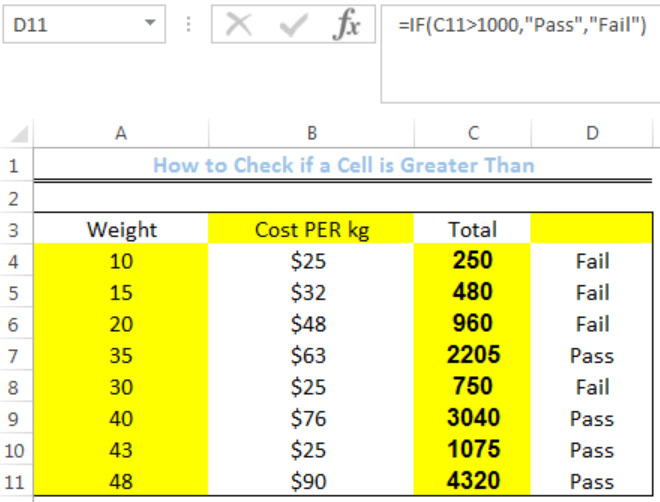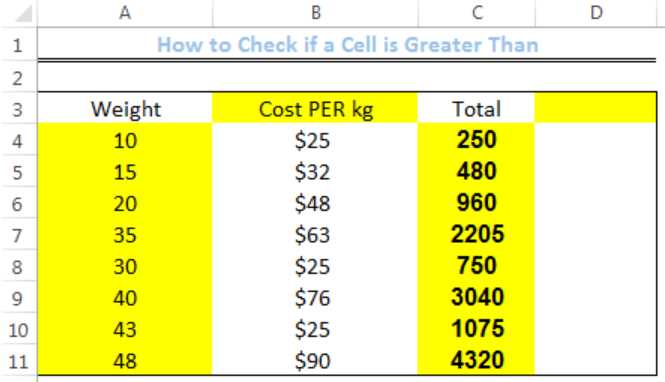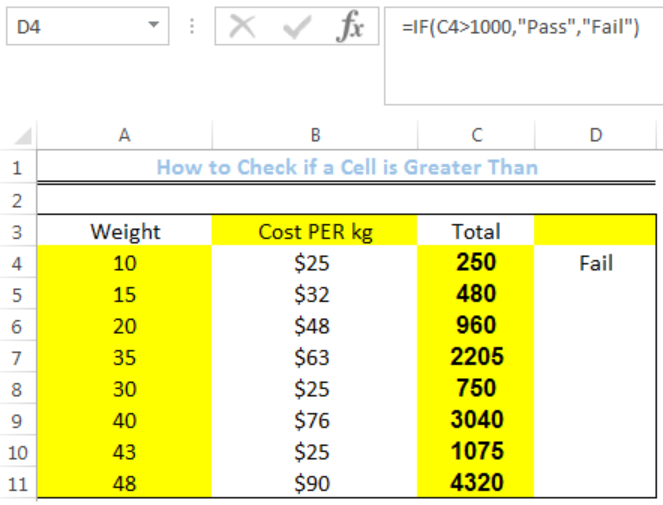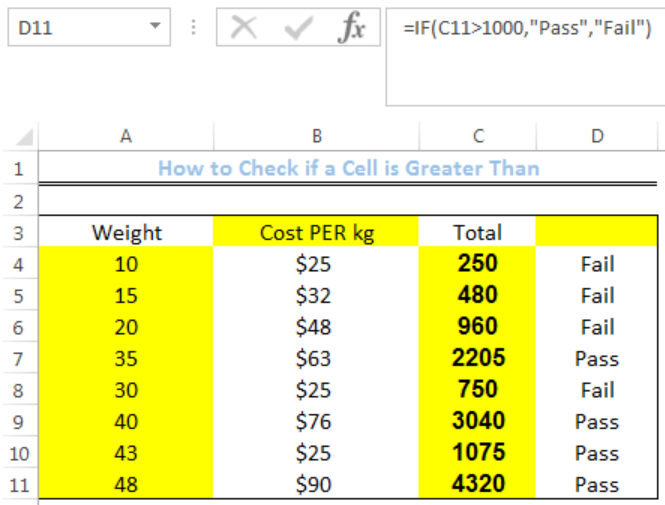Get instant live expert help with Excel or Google Sheets“My Excelchat expert helped me in less than 20 minutes, saving me what would have been 5 hours of work!”

#### Post your problem and you’ll get expert help in seconds.

Your message must be at least 40 characters
Our professional experts are available now. Your privacy is guaranteed.

# How to Check if a Cell is Greater Than

We can use the IF Function to check if a cell has a value that is greater than the specified criteria. The IF function returns TRUE if the condition is met and FALSE if otherwise. The steps below will walk through the process.Figure 1: How to Check if a Cell is Greater Than

## Syntax

`= IF(logical_test,[value_if_true],[value_if_false])`

## Formula

`=IF(C4>1000,"Pass","Fail")`

## Setting up the Data

• We will set up the data by inputting the WEIGHT into Column A
• We will input the cost per kg into Column B
• Column C contains the result of the multiplied values in Column A with Column B
• Column D is where we want the formula to return the result if the cells in Column C are greater than 1000Figure 2: Setting up the Data

## If a Cell is Greater than 1000

• We will click on Cell D4
• We will insert the formula below into the cell
`=IF(C4>1000,"Pass","Fail")`
• We will press the enter keyFigure 3: Result for Cell D4 Indicating it is Less Than 1000

• We will click on Cell D4 again
• We will double-click on the fill handle (the small plus sign at the bottom right of Cell D4) and drag down to copy the formula into the other cellsFigure 4: Result for Column D with the IF function

## Explanation

`=IF(C4>1000,"Pass","Fail")`

The logical test is checked if the value of IS Cell C4 is GREATER THAN 1000. If this is TRUE, the IF Function returns PASS and FAIL if otherwise.

## Instant Connection to an Expert through our Excelchat Service

Most of the time, the problem you will need to solve will be more complex than a simple application of a formula or function. If you want to save hours of research and frustration, try our live Excelchat service! Our Excel Experts are available 24/7 to answer any Excel question you may have. We guarantee a connection within 30 seconds and a customized solution within 20 minutes.

### Did this post not answer your question? Get a solution from connecting with the expert.Another blog reader asked this question today on Excelchat:
Solution examplesCould you help me by explaining the IF function?
Solved by G. L. in 24 minsI have a question about Conditional Formatting. =IF('Sheet1'!A1="X",IF('Sheet1'!B1="Y", TRUE, FALSE),FALSE) the set the cell background to red. I would like to make it so that if Sheet1'!B1="Z" I set the back to blue. How can I achieve this.
Solved by B. F. in 39 minsI need to find out if the 2018 yearly sales goals were met if the yearly sales were \$25,000 or more using an IF logical function and to set the formula to return a value of YES if met, and NO if not
Solved by A. A. in 18 minsI filtered data in column A (it is labeled ID #) of a data set. On my next worksheet, column A is also the ID #, but it is a different data set. I want to filter the ID #'s the same for the two sheets, but how do I do that being that I am working with two different sets of data?
Solved by V. H. in 22 minsi want to create a very simple if function in a cell which is, if one cell is less than 1250, then the automatic value in the current cell is 250, dead easy, for someone who knows! Thank you.
Solved by A. H. in 14 mins## Subscribe to Excelchat.coAnother blog reader asked this question today on Excelchat: